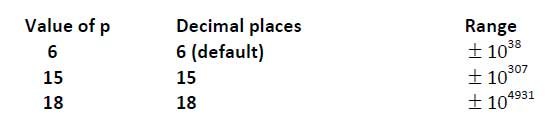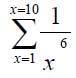# Education is not limited to just classrooms. It can be gained anytime, anywhere... - Ravi Ranjan (M.Tech-NIT)

## Files and Precision

Aims

By the end of this worksheet, you will be able to:

• Read from and write to files
• Use extended precision

Reading from files

In the real world, most of the data we use for our programs will be kept in files. We just need a modification to the read  statement that we are already familiar with to do this.

This program reads 3 numbers from a file called 'mydata.txt' into an array. Use Windows Notepad to create such a file for yourself, or copy the file from mydata.txt which is on the website.

```        program readdata
implicit none
!reads data from a file called mydata.txt
real :: x,y,z
open(10,file='mydata.txt')
read(10,*) x,y,z
print *,x,y,z
end program  readdata
```

The new material here are the lines

```        open(10,file='mydata.txt')
read(10,*) x,y,z
```

The open statement links the file called 'mydata.txt' with an input device numbered 10 (it doesn't have to be 10, it could be any positive integer). To read from device 10, we just use it as the first argument in the read statement.

Exercise 4.1

Use Notepad to create a  file called evenodd.txt. In the file type 10 numbers, one per line. Write a program that reads data from evenodd.txt one line at a time. Check if each number is even or odd and print out a suitable message. One way to check if a number is even or odd is to use the mod intrinsic function, like this…

`                if (mod(num,2)>0) then……`

mod returns the remainder of the first argument divided by the second. If the return value is greater than zero, then the number must be odd.  Check program evenodd.f95 to see if you are correct.

Writing to files

This is a similar idea to reading from files. We need a new statement, though, instead of print, we use write.

```        program io2
!illustrates  writing arrays to files
implicit none
real :: num
integer :: i
open(12,file='myoutput')
do i = 1,100
num = i/3.0
write(12,*) num
end do
print *, 'finished'
end program io2
```

Exercise 4.2

Write a program which reads in numbers from a file one at a time. If the number is positive, it should  store it in a file called 'positive.txt' and negative numbers in a file called 'negative.txt'.

Extending the precision

So far, we have used two types of variables, real and integer. The problem so far, as you will have noticed on output, is that we are extremely limited by the number of significant digits that are available for computation. Clearly, when we are dealing with iterative processes, this will lead rapidly to errors. We can, however, extend the precision available from the single precision default, which gives us 6 figure decimal precision to 15 figures by using a new specification for real numbers.

```        program extended
implicit none
integer, parameter  :: ikind=selected_real_kind(p=15)
real (kind=ikind)  :: sum,x
integer :: i
sum=0.0
do i=1,100
x=i
sum = sum +  1.0/(x**6)
end do
print *, sum
end program  extended
```

produces the following output:

```        1.01734306196
```

Don't be put off by the odd looking code. In practice, the way of setting up this extended precision, is pretty much the same for every program.

We state the precision we want by the argument p

```
integer, parameter ::  ikind=selected_real_kind(p=15)
```

in this case, 15 decimal places. ikind is a new data type – a parameter. FORTRAN returns a value to the parameter ikind that will be adequate to provide 15 digit precision. This code will work on any machine irrespective of the architecture.

We declare that the variables are using extended precision by

```        real (kind=ikind) ::  sum,x
```

Valid values for are 6, 15 and 18. The default value for is 6. If you ask for more precision than 18 digits, the compiler will complain with an error message.  Try changing the values of  and see what effect this has on the output.

The trouble with PRINT is that the programmer has no control over the number of digits output irrespective of the selected precision .

Later on we'll come back to this when we learn about the WRITE statement, and output formatting.

Note Unlike variables, parameters may not change once they are declared.

If we want to use constants in a program that uses extended precision, we have to tell FORTRAN that they are also extended precision explicitly. This leads to the rather strange syntax you can see in the following program.

```   program extendedconstants
!demonstrates use of extended precision
implicit none
integer, parameter ::  ikind=selected_real_kind(p=18)
real (kind=ikind) :: val,x,y
val=10/3
print*,val                  !10/3 calculated as  integer  - wrong!
x=10.0
y=3.0
val=x/y              !x/y assigned to extended  precision - right!
print*,val
val=10.0_ikind/3              !extend precision constant -  right!
print*,val
val=10.0/3.0                              !real constants - wrong!
print*,val
val = .12345678901234567890               !real constants - wrong!
print *, val
val = .12345678901234567890_ikind   !ext precision consts - right!
print *, val
end program  extendedconstants
```

You should run this program for yourself and think carefully about its implications. This program demonstrates how easy it is to get calculations wrong.  I’ll leave this to you to experiment to ensure that you fully understand the importance of properly declaring variables and the use of constants in FORTRAN programming. A systematic approach to your programming will reduce the risk of errors as will running programs with test data that have known solutions so that you can confirm that your program is error free.

Magnitude limitations

We have already observed that there is a limitation of the accuracy with which we can do calculations in FORTRAN (and indeed, any, computer language). There are also limitations on the magnitude of a number. The various magnitude and precision limits are summarized in the following table:

####Exercise 5.3

To illustrate these limits copy file  magnitude.f95  and run the program. Take a while to get a feel for what is going on. Try inputting various values for the variable maxpower (eg 400). Can you confirm that the table above is correct?

One interesting construct is

```	print *,i,2.0_ikind**i
```

Here, we are telling the compiler that the real constant 2.0 is also using extended precision. Check what happens if you select extended precision (option 3) and enter a value of maxpower of 400. See what happens  if you rewrite the line to be

`	print *,i,2.0**i`

Run the program again and enter the same values. Can you explain what is going on?

Convergence – exiting loops  on a condition

In the program extended.f95, we found the sum ofIt is useful to determine at what point such sums converge to a steady value – otherwise we may make arbitrary assumptions about the summation range. Clearly, a point will be reached, within the precision range that can be handled on our computer, that the termwill be too small to contribute to the sum. At this point we should exit the loop otherwise the program will do more computation than is required.

One way to do this is to compare the value of the variable sum with its previous value, and if the difference between the two is very small, then exit the loop.

```  program  whileloop
implicit none
integer, parameter ::  ikind=selected_real_kind(p=15)
real (kind=ikind) ::  sum,previoussum,x,smallnumber,error
integer :: i
sum = 0.0
previoussum = 0.0
smallnumber = 10.0_ikind**(-15.0)
do i=1,1000
x=i
sum = sum + 1.0 /(x**6)
error=abs(sum-previoussum)
if  (error```

IMPORTANT NOTE

In the real world, we have to make choices about the amount of precision we need to work to. It is pointless asking for 15 digits of precision if, for example, we can only take a measurement to + or – 1% accuracy!

It is not necessary  to always use a loop counter in a do loop.  If we don't actually specify a counter, the program will loop forever. Constructs like this are OK:

```  	smallnumber = .0000001_ikind
do
print *, 'enter a  positive number '
read *, number
if (number <=  smallnumber) exit
end do
```

The disadvantage is that, if you get the code wrong, you run the risk of the program looping forever – generally it's safer to use a loop counter!